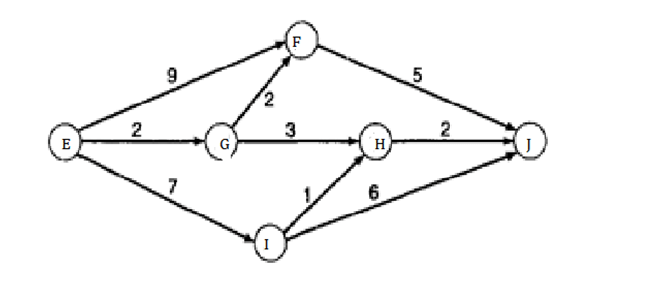Directions (Qs.1-5): Answer the questions on the basis of the information given below.
A significant amount of traffic flows from point E to point J in the one-way street network shown below. Points F, G, H and I are junctions in the network, and the arrows mark the direction of traffic flow. The fuel cost in rupees for travelling along a street is indicated by the number adjacent to the arrow representing the street.Motorists travelling from point E to point J would obviously take the route for which the total cost of travelling is the minimum. If two or more routes have the same least travel cost, then motorists are indifferent between them. Hence, the traffic gets evenly distributed among all the least cost routes.
The government can control the flow of traffic only by levying appropriate toll at each junction. For example, if a motorist takes the route E-F-J (using junction F alone), then the total cost of travel would be Rs. 14. (i.e., Rs. 9 + Rs 5) plus the toll charged at junction F.

Question 1: If the government wants to ensure that the traffic at S gets evenly distributed along streets from E to F, from E to G, and from E to I, then a feasible set of toll charged (in rupees) at junctions F,G,H and I, respectively to achieve this goal is
(a) 0, 4, 3, 2
(b) 1, 5, 3, 3
(c) 0, 5, 2, 2
(d) 1, 5, 3, 2
(e) 0, 5, 4, 1

Question 2 : If the government wants to ensure that all motorists travelling from E to J pay the same amount (fuel costs and toll combined) regardless of the route they choose and the street from G to H is under repairs (and hence unusable), then a feasible set of toll charged (in rupees) at junctions F,G, H and I respectively to achieve this goal is :
(a) 2, 5, 3, 2
(b) 0, 5, 3, 1
(c) 1, 5, 3, 0
(d) 2, 3, 5, 1
(e) 1, 3, 5, 1

Question 3: If the government wants to ensure that all routes from E to J get the same amount of traffic, then a feasible set of toll charged (in rupees) at junctions F,G,H and I respectively to achieve this goal is
(a) 0, 5, 4, 1
(b) 0, 5, 2, 2
(c) 1, 5, 4, 2
(d) 1, 5, 3, 2
(e) 1, 5, 3, 3

Question 4:  If the government wants to ensure that no traffic flows on the street from I to J, while equal amount of traffic flows through junctions F and H, then a feasible set of toll charged (in rupees) at junctions F,G,H and I respectively to achieve this goal is
(a) 1, 5, 3, 3
(b) 0, 5, 2, 3
(c) 1, 5, 4, 2
(d) 1, 4, 4, 3
(e) 0, 5, 2, 2

Question 5: The government wants to devise a toll policy such that the total cost to the commuters per trip is minimized. The policy should also ensure that not more than 70% of the total traffic passes through junction G. The cost incurred by the commuter travelling from point E to point J under this policy will be
(a) Rs. 7
(b) Rs. 9
(c) Rs. 10
(d) Rs. 13
(e) Rs. 14

Traffic among the different paths will be evenly distributed if the cost of travel (fuel cost plus toll charges) is same through all the given routes. Option (d) gives the cost of travel through all the routes as same.

F= 1, G=5, H = 3, I = 2

EF: EFJ = 9 + 1 +5 = Rs.15

E⇒G: EGFJ = 2 + 5 + 2 + 1 +5 = Rs.15

EGHJ=2 + 5+3+3 + 2 = Rs.15

E⇒I: EIHJ = 7 + 2 + 1 +3 + 2 = Rs.15

EIJ= 7 + 2 + 6 = Rs.15

It is given in the question that path GH is under repair, hence, available paths to reach from E to J are EGHJ, EFJ, EIHJ and EIJ. Now, using options, we find that option (b) gives the same cost of travel from S to T.

F=0, G = 5, H = 3, I = 1

EGFJ = 2 + 5 + 2 + 0 + 5 = Rs.14

EFJ = 9 + 0 + 5=Rs.14

EIHJ = 7 + l + l + 3 + 2 = Rs.14

EIJ = 7 + l + 6 = Rs.14

For option (d), we have

F= 1, G=5, H = 3, I = 2

EFJ = 9 + l + 5=Rs.15

EGFJ = 2 + 5 + 2 + 1 +5 = Rs.15

EIJ = 7 + 2 + 6 = Rs.15

EIHJ = 7 + 2 + l + 3 + 2 = Rs.15

EGHJ = 2 + 5 + 3+3 + 2 = Rs.15

Traffic from I to J is restricted, hence, available routes through F and H are EFJ,EGFJ,EGHJ,EIHJ. Option (e) gives

the same cost of travel.

EFJ = 9 + 0 + 5=Rs.14

EGFJ = 2 + 5 + 2+0 + 5 = Rs.14

EGHJ =2 + 5 + 3+2 + 2 = Rs.14

EIHJ = 7 + 2 + l + 2 + 2 = Rs.14